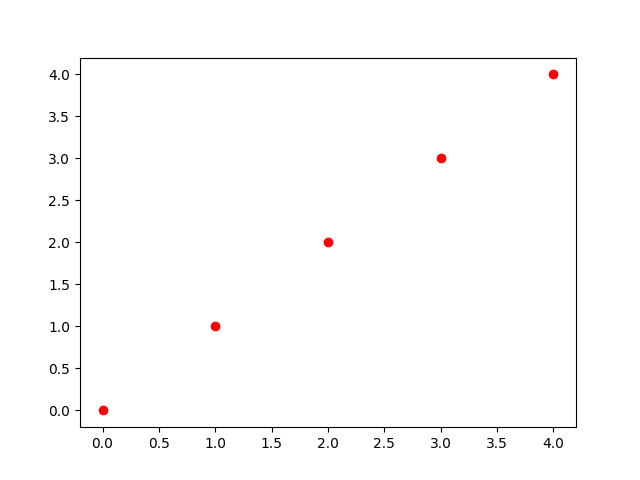# Displaying a custom label for each individual point¶

mpldatacursor’s `point_labels` functionality can be emulated with an event handler that sets the annotation text with a label selected from the target index.```import matplotlib.pyplot as plt
import mplcursors
import numpy as np

labels = ["a", "b", "c", "d", "e"]
x = np.array([0, 1, 2, 3, 4])

fig, ax = plt.subplots()
line, = ax.plot(x, x, "ro")
mplcursors.cursor(ax).connect(